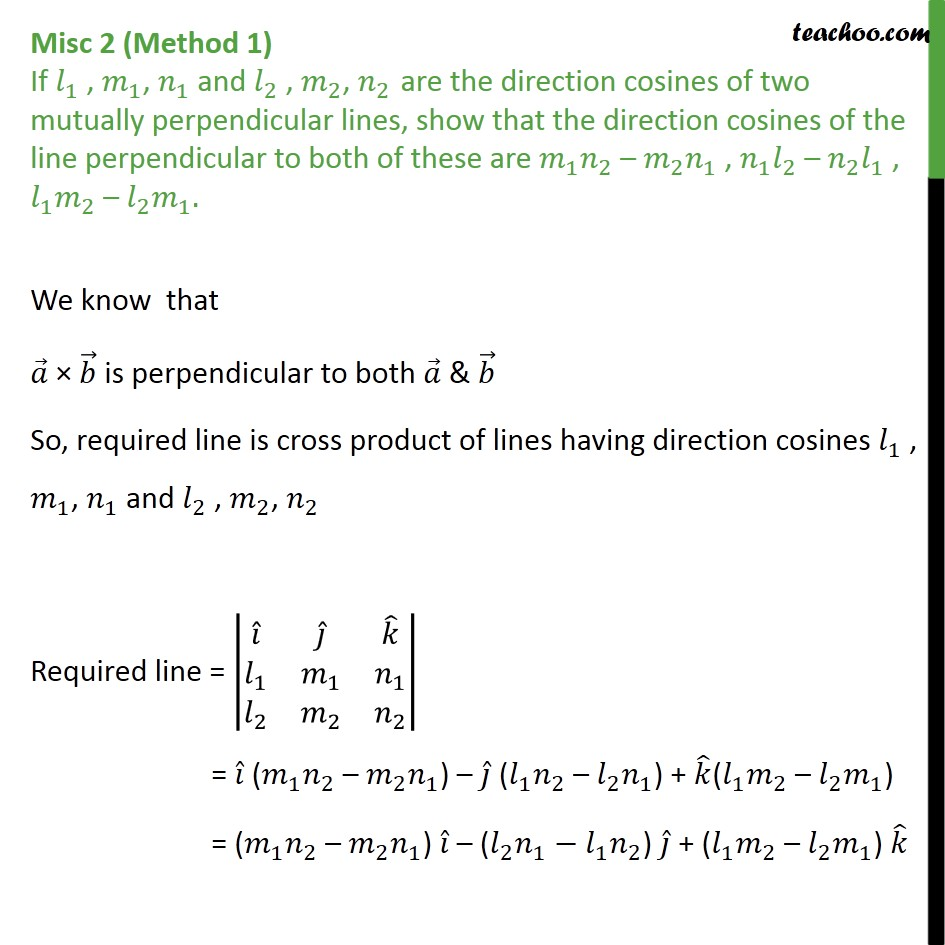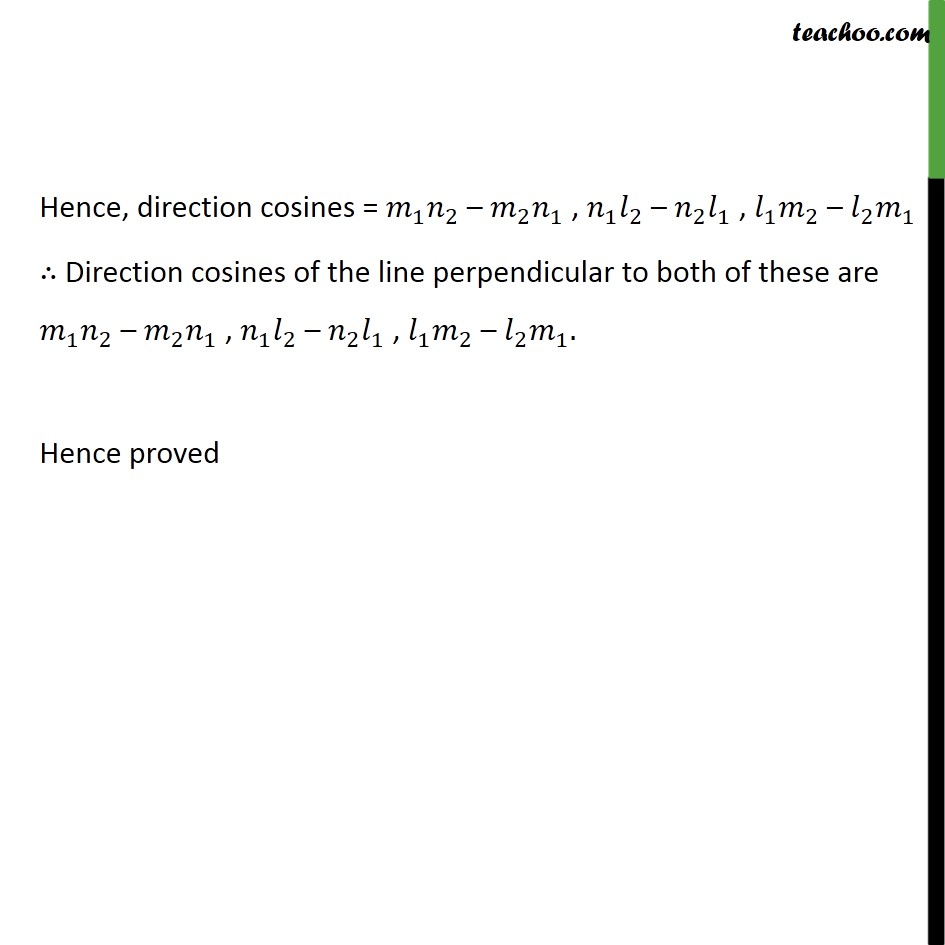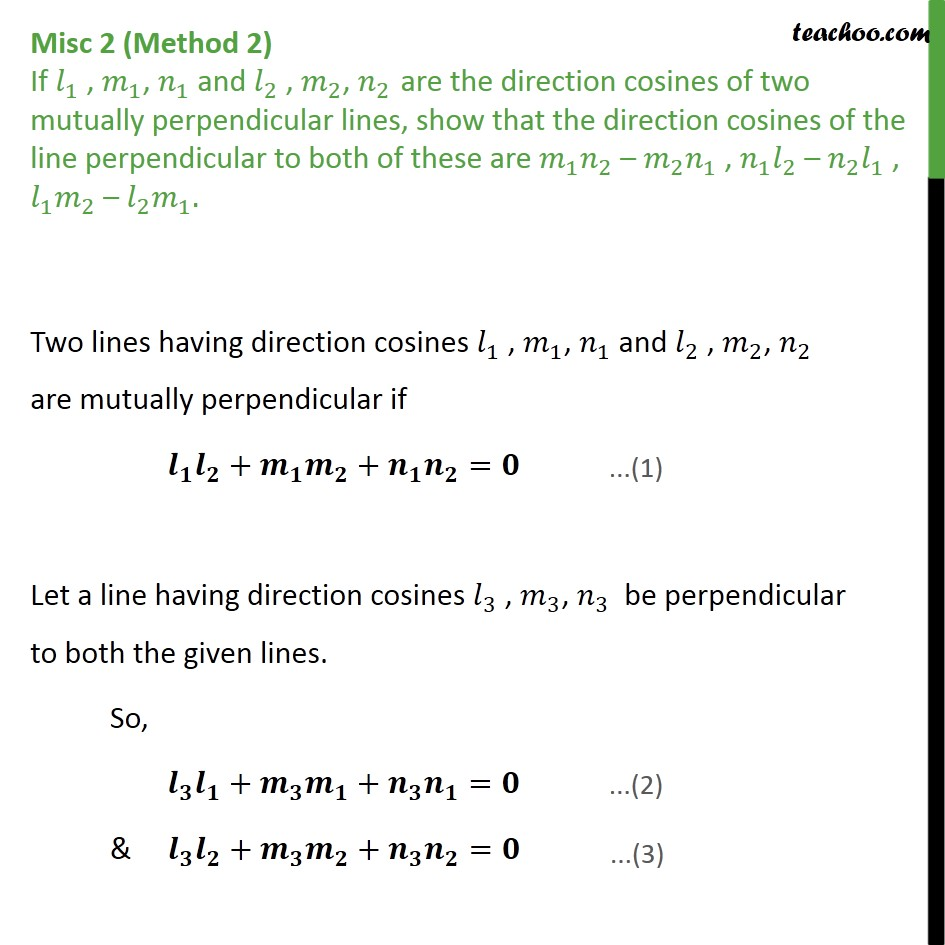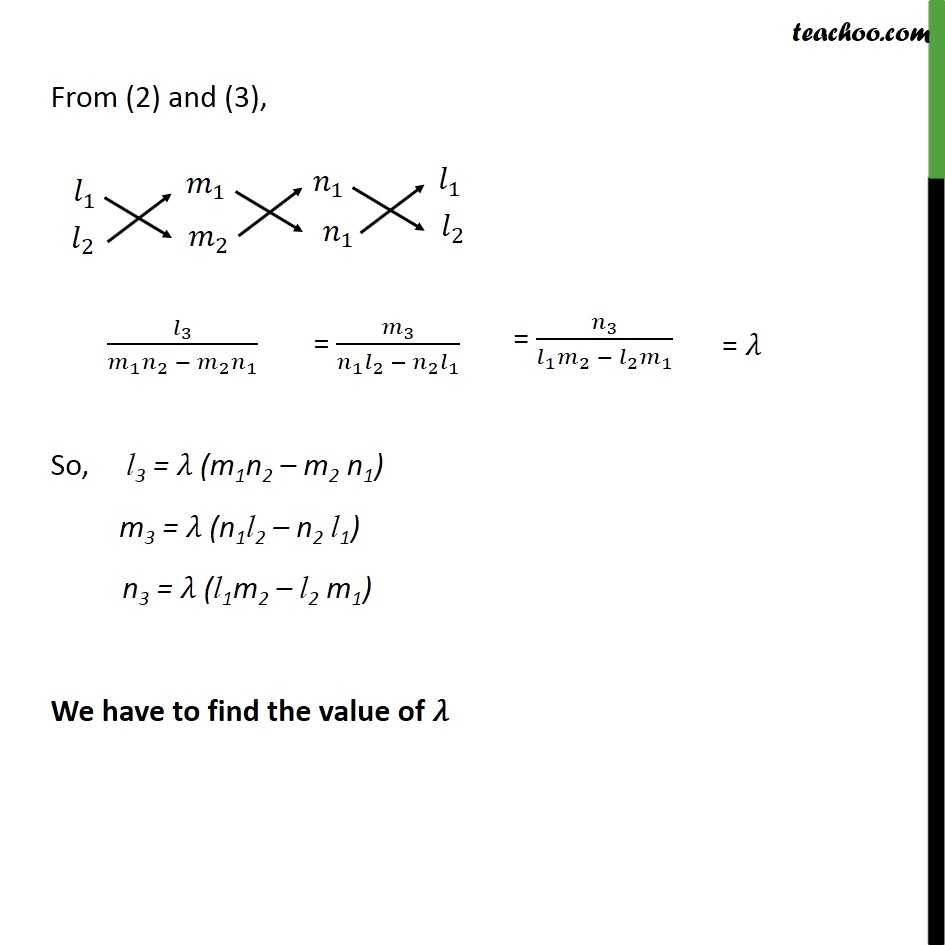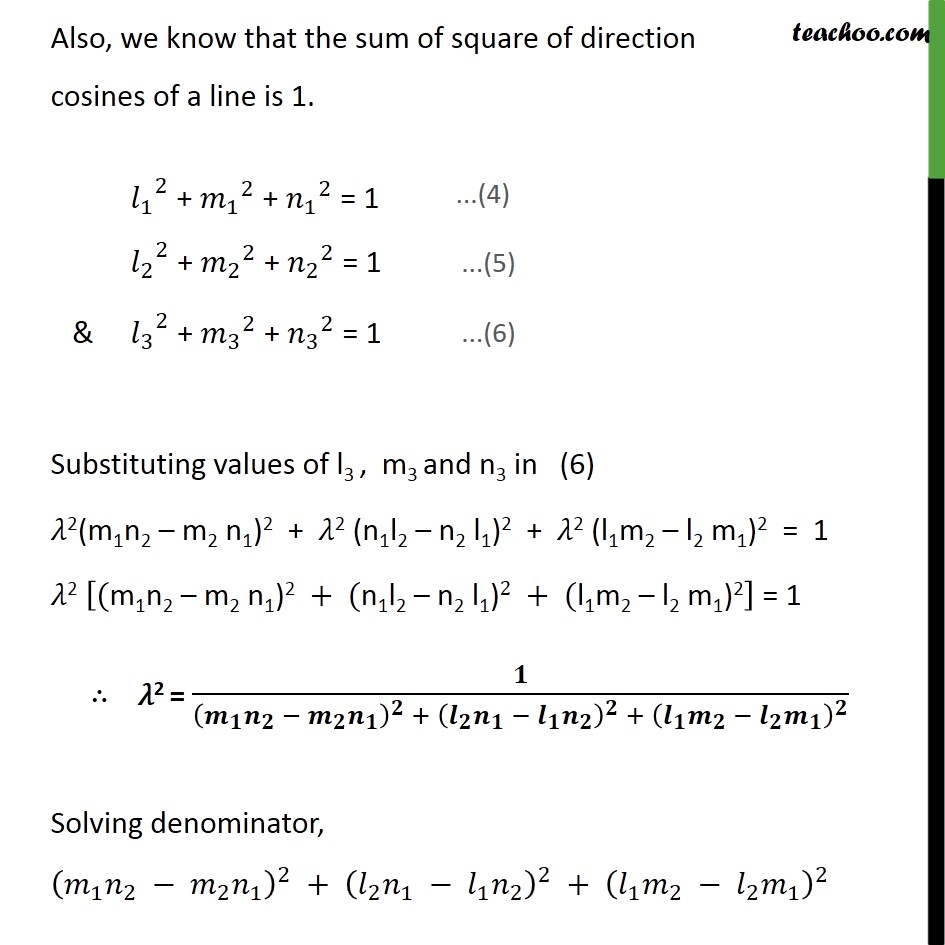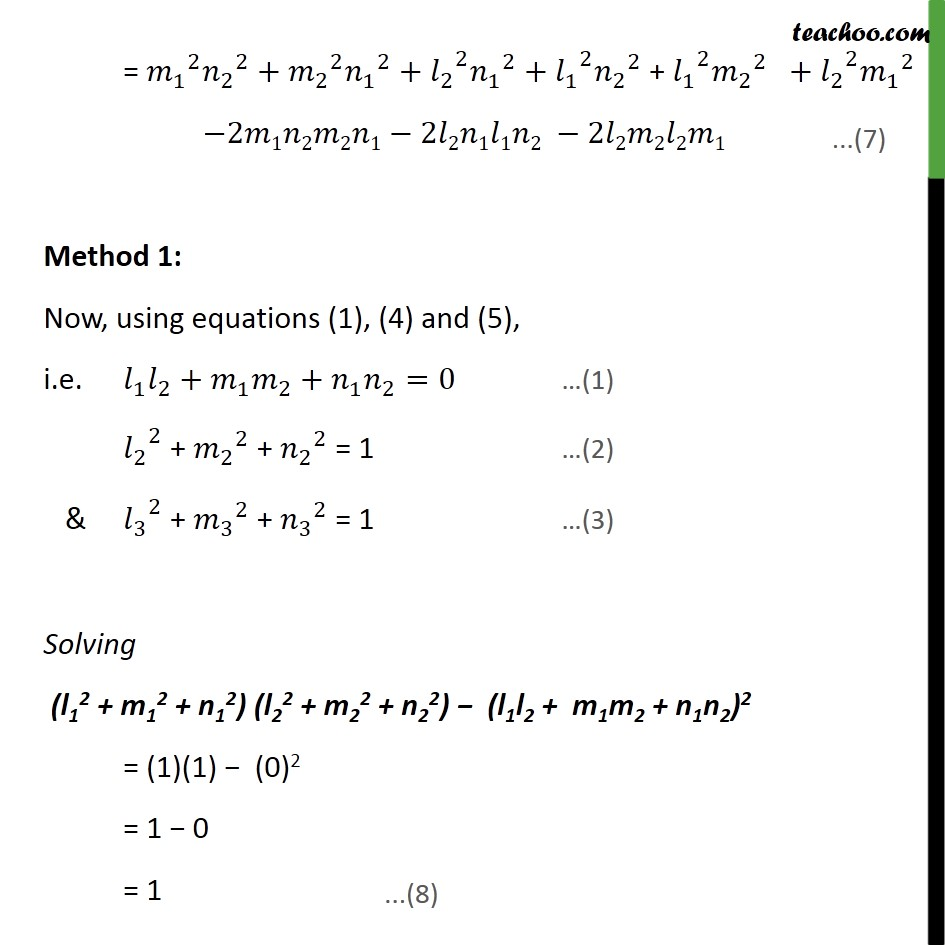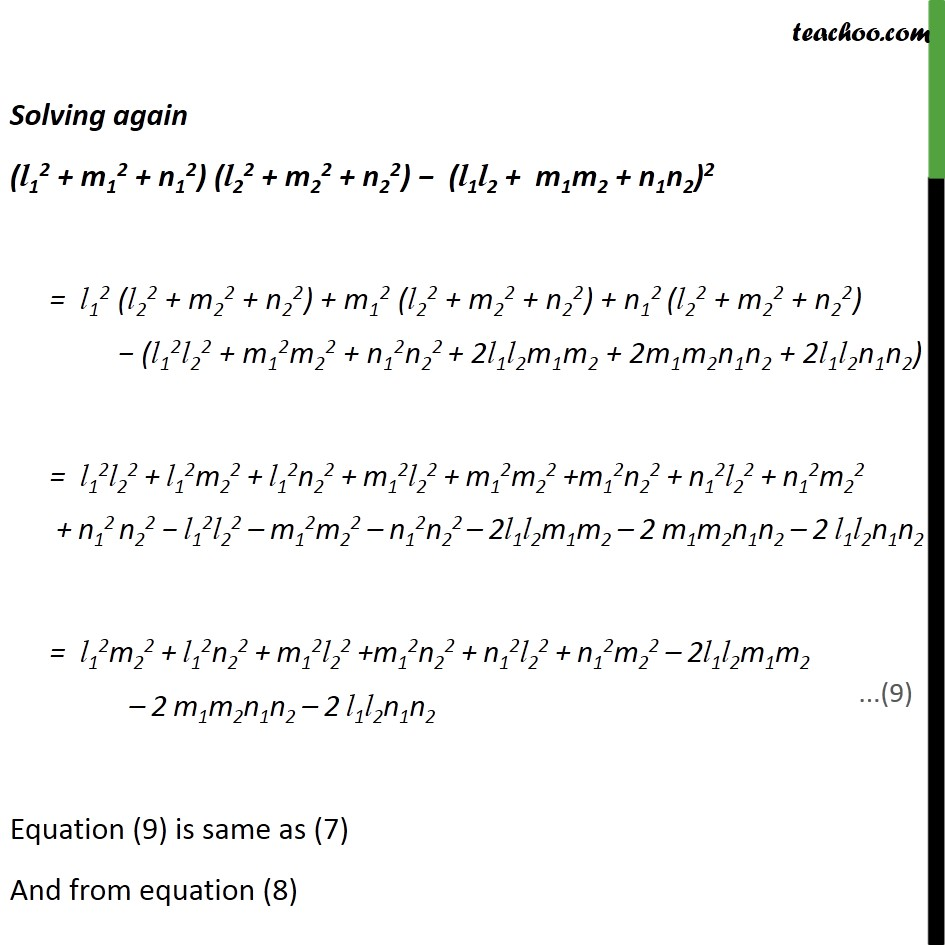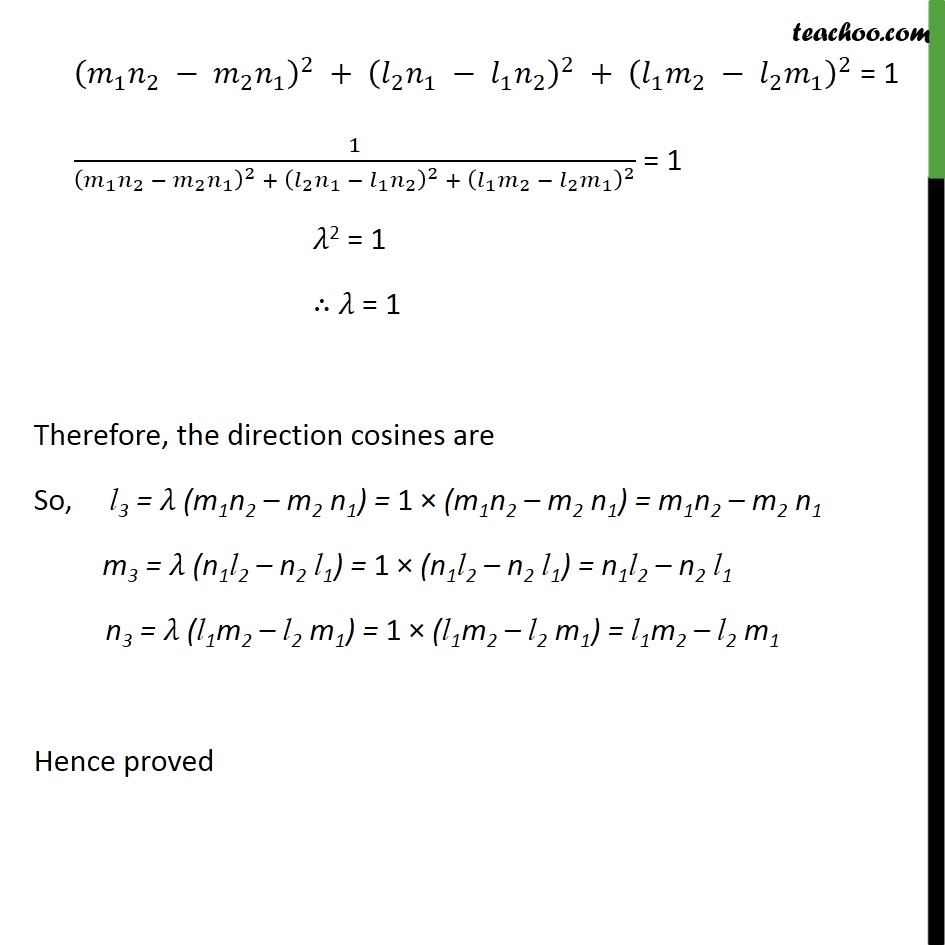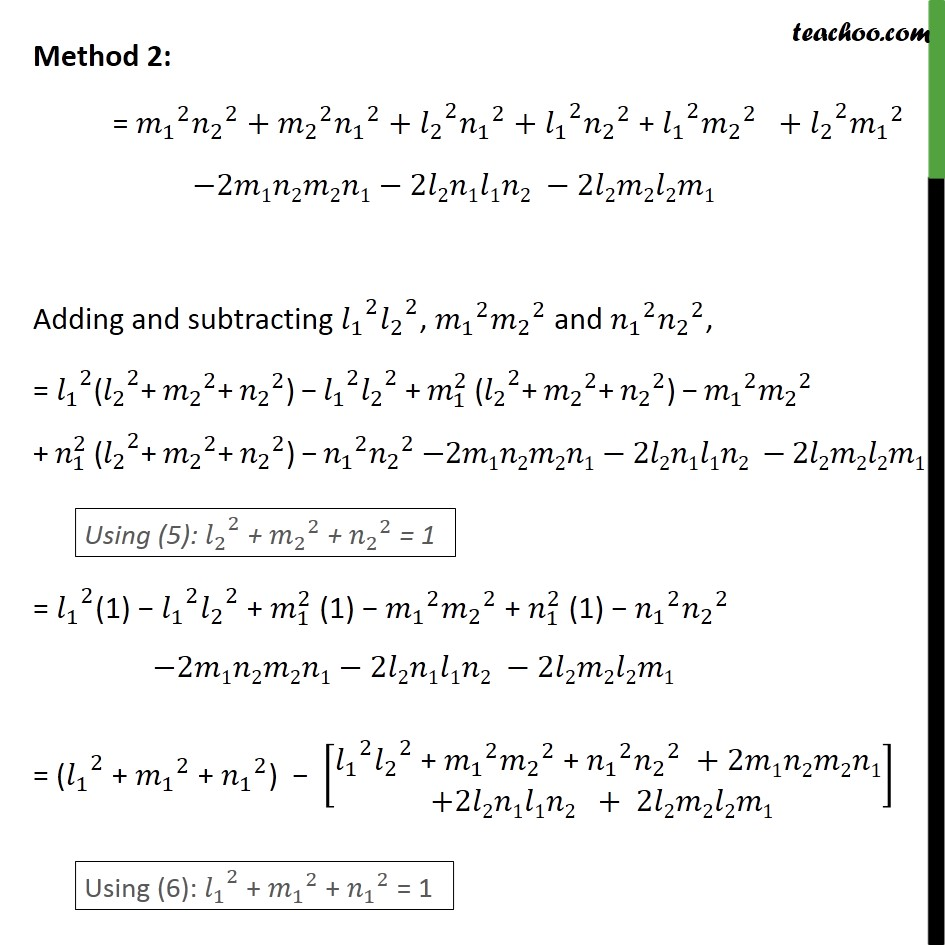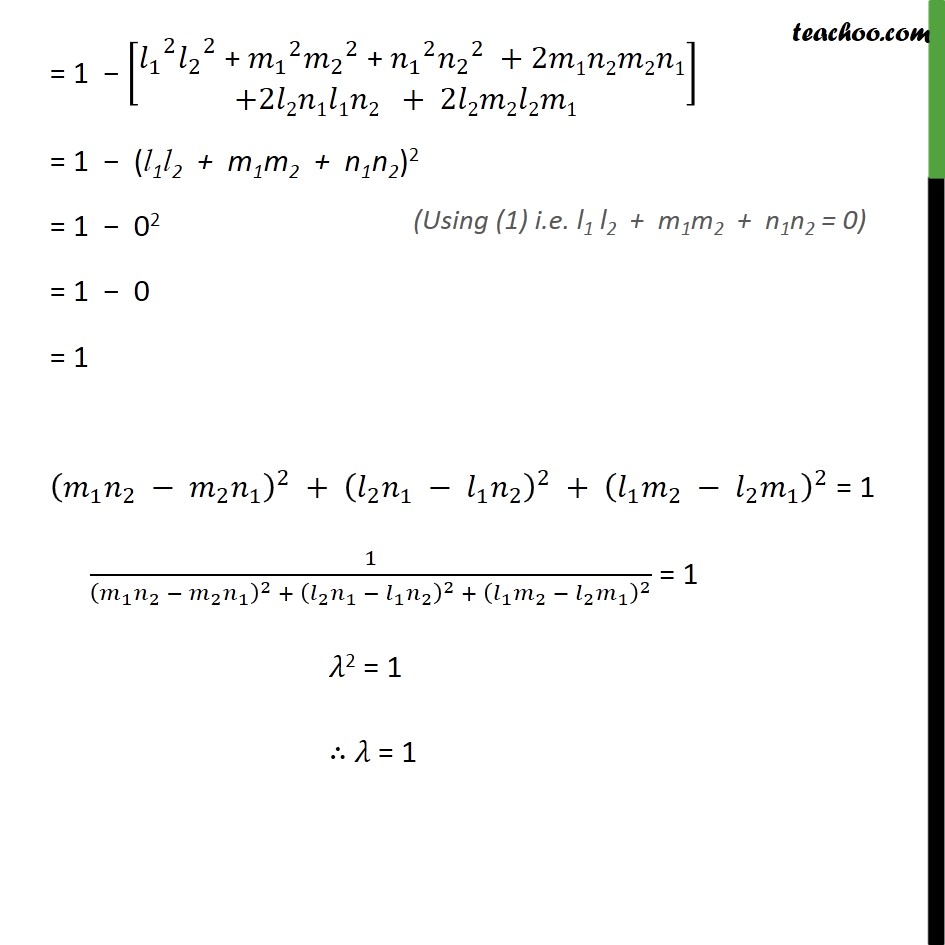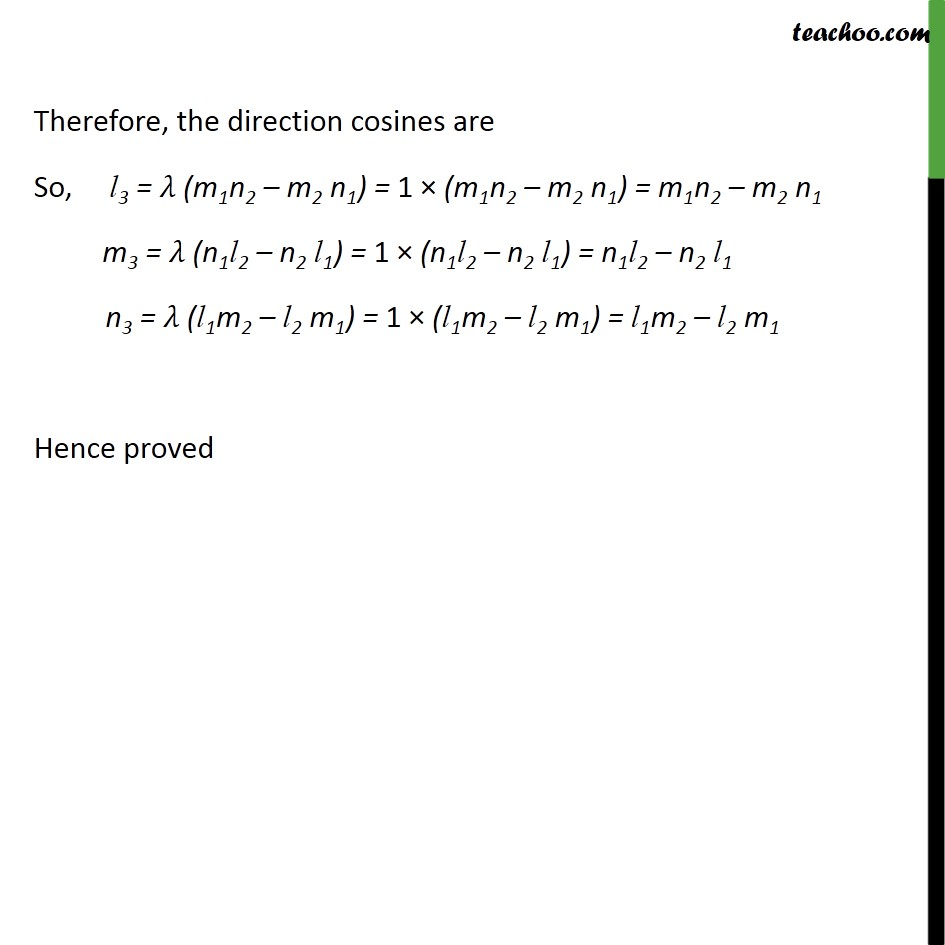1. Chapter 11 Class 12 Three Dimensional Geometry
2. Serial order wise
3. Miscellaneous

Transcript

Misc 2 (Method 1) If 1 , 1 , 1 and 2 , 2 , 2 are the direction cosines of two mutually perpendicular lines, show that the direction cosines of the line perpendicular to both of these are 1 2 2 1 , 1 2 2 1 , 1 2 2 1 . We know that is perpendicular to both & So, required line is cross product of lines having direction cosines 1 , 1 , 1 and 2 , 2 , 2 Required line = 1 1 1 2 2 2 = ( 1 2 2 1 ) ( 1 2 2 1 ) + ( 1 2 2 1 ) = ( 1 2 2 1 ) ( 2 1 1 2 ) + ( 1 2 2 1 ) Hence, direction cosines = 1 2 2 1 , 1 2 2 1 , 1 2 2 1 Direction cosines of the line perpendicular to both of these are 1 2 2 1 , 1 2 2 1 , 1 2 2 1 . Hence proved Misc 2 (Method 2) If 1 , 1 , 1 and 2 , 2 , 2 are the direction cosines of two mutually perpendicular lines, show that the direction cosines of the line perpendicular to both of these are 1 2 2 1 , 1 2 2 1 , 1 2 2 1 . Two lines having direction cosines 1 , 1 , 1 and 2 , 2 , 2 are mutually perpendicular if + + = Let a line having direction cosines 3 , 3 , 3 be perpendicular to both the given lines. So, + + = & + + = From (2) and (3), So, l3 = (m1n2 m2 n1) m3 = (n1l2 n2 l1) n3 = (l1m2 l2 m1) We have to find the value of Also, we know that the sum of square of direction cosines of a line is 1. 1 2 + 1 2 + 1 2 = 1 2 2 + 2 2 + 2 2 = 1 & 3 2 + 3 2 + 3 2 = 1 Substituting values of l3 , m3 and n3 in (6) 2(m1n2 m2 n1)2 + 2 (n1l2 n2 l1)2 + 2 (l1m2 l2 m1)2 = 1 2 (m1n2 m2 n1)2 + (n1l2 n2 l1)2 + (l1m2 l2 m1)2 = 1 2 = + + Solving denominator, 1 2 2 1 2 + 2 1 1 2 2 + 1 2 2 1 2 = 1 2 2 2 + 2 2 1 2 + 2 2 1 2 + 1 2 2 2 + 1 2 2 2 + 2 2 1 2 2 1 2 2 1 2 2 1 1 2 2 2 2 2 1 Method 1: Now, using equations (1), (4) and (5), i.e. 1 2 + 1 2 + 1 2 =0 (1) 2 2 + 2 2 + 2 2 = 1 (2) & 3 2 + 3 2 + 3 2 = 1 (3) Solving (l12 + m12 + n12) (l22 + m22 + n22) (l1l2 + m1m2 + n1n2)2 = (1)(1) (0)2 = 1 0 = 1 Solving again (l12 + m12 + n12) (l22 + m22 + n22) (l1l2 + m1m2 + n1n2)2 = l12 (l22 + m22 + n22) + m12 (l22 + m22 + n22) + n12 (l22 + m22 + n22) (l12l22 + m12m22 + n12n22 + 2l1l2m1m2 + 2m1m2n1n2 + 2l1l2n1n2) = l12l22 + l12m22 + l12n22 + m12l22 + m12m22 +m12n22 + n12l22 + n12m22 + n12 n22 l12l22 m12m22 n12n22 2l1l2m1m2 2 m1m2n1n2 2 l1l2n1n2 = l12m22 + l12n22 + m12l22 +m12n22 + n12l22 + n12m22 2l1l2m1m2 2 m1m2n1n2 2 l1l2n1n2 Equation (9) is same as (7) And from equation (8) 1 2 2 1 2 + 2 1 1 2 2 + 1 2 2 1 2 = 1 1 1 2 2 1 2 + 2 1 1 2 2 + 1 2 2 1 2 = 1 2 = 1 = 1 Therefore, the direction cosines are So, l3 = (m1n2 m2 n1) = 1 (m1n2 m2 n1) = m1n2 m2 n1 m3 = (n1l2 n2 l1) = 1 (n1l2 n2 l1) = n1l2 n2 l1 n3 = (l1m2 l2 m1) = 1 (l1m2 l2 m1) = l1m2 l2 m1 Hence proved Method 2: = 1 2 2 2 + 2 2 1 2 + 2 2 1 2 + 1 2 2 2 + 1 2 2 2 + 2 2 1 2 2 1 2 2 1 2 2 1 1 2 2 2 2 2 1 Adding and subtracting 1 2 2 2 , 1 2 2 2 and 1 2 2 2 , = 1 2 ( 2 2 + 2 2 + 2 2 ) 1 2 2 2 + 1 2 ( 2 2 + 2 2 + 2 2 ) 1 2 2 2 + 1 2 ( 2 2 + 2 2 + 2 2 ) 1 2 2 2 2 1 2 2 1 2 2 1 1 2 2 2 2 2 1 = 1 2 (1) 1 2 2 2 + 1 2 (1) 1 2 2 2 + 1 2 (1) 1 2 2 2 2 1 2 2 1 2 2 1 1 2 2 2 2 2 1 = ( 1 2 + 1 2 + 1 2 ) 1 2 2 2 + 1 2 2 2 + 1 2 2 2 +2 1 2 2 1 +2 2 1 1 2 + 2 2 2 2 1 = 1 1 2 2 2 + 1 2 2 2 + 1 2 2 2 +2 1 2 2 1 +2 2 1 1 2 + 2 2 2 2 1 = 1 (l1l2 + m1m2 + n1n2)2 = 1 02 = 1 0 = 1 1 2 2 1 2 + 2 1 1 2 2 + 1 2 2 1 2 = 1 1 1 2 2 1 2 + 2 1 1 2 2 + 1 2 2 1 2 = 1 2 = 1 = 1 Therefore, the direction cosines are So, l3 = (m1n2 m2 n1) = 1 (m1n2 m2 n1) = m1n2 m2 n1 m3 = (n1l2 n2 l1) = 1 (n1l2 n2 l1) = n1l2 n2 l1 n3 = (l1m2 l2 m1) = 1 (l1m2 l2 m1) = l1m2 l2 m1 Hence proved

Miscellaneous